Extinction Shift Principle© since April 15, 2001 on the web

 Astrophysical Evidence Clearly Shows A Direct Interaction between Gravitation and Electromagnetism Occurs Nowhere in Plasma-Free Vacuum Space

Updated: July 29, 2018

 The Abbreviated Details ABSTRACT Microwaves Found to Deflect only at Minimum Impact Parameter when Propagated in the Plasma Limb of the Sun

This work reveals modern astrophysical evidence that clearly counters all that is being published in the literature and that is currently being taught in academia; namely that which pertains to the effects of gravitation and electromagnetism and whether these effects pertain to a direct- or an indirect interaction between gravitation and electromagnetism.
Three mainly important topics are elucidated here:
 1. A Century of Observations  on Gravitational Lensing 2. The Fundamentals of Gravitational Lensing 3. The Flawed Teaching Misinterpretation  of the Observations Misrepresentation of the Correct Fundamentals A Flawed Tool that does not represent the True Fundamentals
All of the bullets listed under 3. have led to the acceptance of the false teachings, very bad referees and to the publication of some bad papers and bad textbooks

The Observations The Fundamentals The Flawed Teaching
Nearly a century of observations on what is understood to be gravitational lensing is often supported by a misinterpretation of the observations coupled with a deviation from the profound Fundamentals of Mathematical Physics that correctly represents the Physics of the phenomenon being observed. The concept of what is understood to be gravitational lensing is continually worsening by the false teachings, incorrectly claiming it to be the true fundamentals. We present here a correct understanding of the Astrophysics along with clear graphical illustrations. A clear understanding of the fundamentals of Gravitational lensing as is predicted by General Relativity is bounced off of the clear observational evidence and the profound Fundamentals of Mathematical Physics. It is herewith shown that the predictions of the Light Bending Rule of General Relativity are clearly violated in the plasma-free vacuum space, and there is enormous evidence for it everywhere in empty vacuum space. Clear observational evidence reveals that a direct interaction between gravitation and electromagnetism does not take place anywhere in plasma-free vacuum space.  A frequently used, poorly designed geometrical lensing tool commonly referred to as the  "Ray Geometry Technique" is found to lead to gross misinterpretations of the observations dealing with gravitational lensing galaxies. These tools are too often used by researchers who base their findings on what they incorrectly claim to be gravitational lensing galaxies. Many of these claims turn out be "False Alarms". These claims can be easily debunked simple by observing the so-called lensing galaxies in a different spectra or range of frequencies, i.e., in UV or in IR. By doing so, the lensing galaxy will appear to have totally different features, rendering many of the claims as "False Alarms".

 I. THE OBSERVATIONS

Microwave Deflections at the Solar Plasma Limb

Findings Clearly Supported by Astrophysical Evidence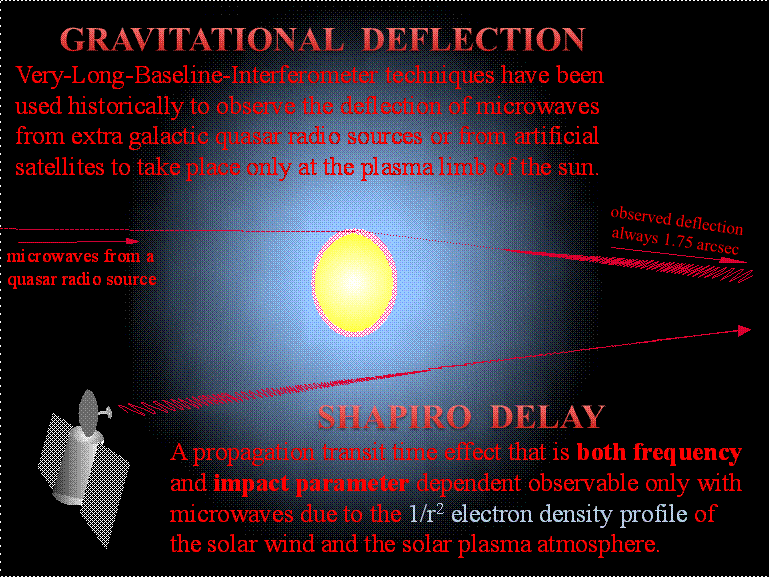Microwaves from quasar radio sources and from artificial satellites are found to deflect only at the plasma limb of the sun, precisely at 1.752 arcsec and most specifically only at a minimum impact parameter corresponding to the solar plasma limb. A large number of experiments have already taken place where researchers have used Mariner Satellites in conjunction with a number of U.S. spacecraft launched by JPL and NASA Mariner missions designed to record radio pulsar signals from extra galactic radio pulsar sources. The emissions from these sources are in the microwave bands. As already stated, a history of VLBI measurements have recorded the gravitational deflections at the solar plasma-limb of microwaves, recording the deflections to be exactly 1.752 arcsec deviating from the initial path of the microwaves emitted from chosen extra galactic quasar radio sources. At astronomical distances the rays of the microwaves from single sources are virtually parallel with one another as can be detected by modern astronomical instruments as depicted in the Figure above. As the VLBI detectors involving microwave antennas stationed on Earth based observatories move along an Earth orbital path, the gravitational deflection of the rays of microwaves are recorded by researchers conducting these experiments, noting the events of gravitational deflections at the solar plasma limb. Historically, the rays of microwaves are observed to deflect only at the angle of 1.752 arcsec, which corresponds to an impact parameter of exactly one solar radii or a distance of R = 695,500 km from the center of the sun. Only at this impact parameter will any star of one solar mass and one solar radius theoretically deflect a ray of microwaves according to the light bending rule of General Relativity at the angle of 1.752 arcsec. If the light bending rule of General Relativity were actually valid then a gravitational deflection of microwaves should also deflect clearly above the plasma limbs of the sun and stars and be observable also at other impact parameters, namely, at the impact parameter of 2R to reveal a gravitational deflection of exactly 0.875 arcsec, at 3R to reveal a gravitational deflection of exactly 1/3 of 1.752 arcsec, etc., etc. Historically, deflections of microwaves are yet to be recorded at impact parameters greater than that of the solar radius R, permitting the observation of deflections at angles less than that of 1.752 arcsec.

References

1. Lebach, D. E. et al. "Measurement of the Solar Gravitational Deflection of Radio Waves Using Very-Long-Baseline Interferometry ", Phys.Rev.Lett, 75 (1995), pp. 1439-1442

2. Counselman, C.C. et al., "Solar Gravitational Deflection of Radio Waves Measured by Very-Long-Baseline Interferometry",  Phys.Rev.Lett. 33 (1974) 1621-1623

3. Fomalont, E. B., et al., "Measurements of the Solar Gravitational Deflection of Radio Waves in Agreement with General Relativity", Phys.Rev.Lett. 36 (1976) 1475-1478

The Observations versus General Relativity

Finding Clearly Supported by Astrophysical Evidence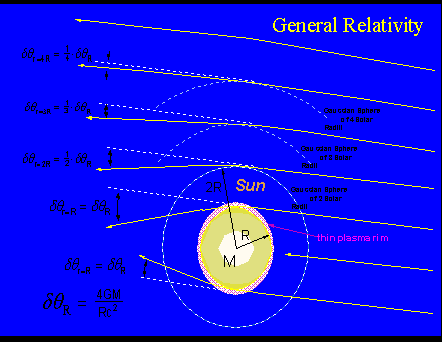The Prediction of General Relativity and the Observations Alternately A direct interaction between Gravitation and Electromagnetism occurs nowhere in plasma-free vacuum space

 The Observations at Sagittarius A*, the Galactic Center Finding Clearly Supported by Astrophysical Evidence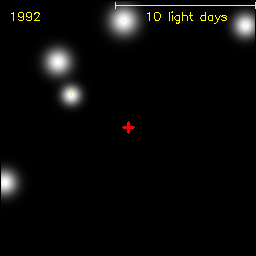Undistorted time resolved images of stellar objects orbiting about Sagittarius A* were collected from 1992 to 2006 by Max-Planck-Institut für extraterrestrische Physik.  For details: http://www.mpe.mpg.de A Star orbits a Black Hole as researchers believe occurs at the site of Sagittarius A* An Animation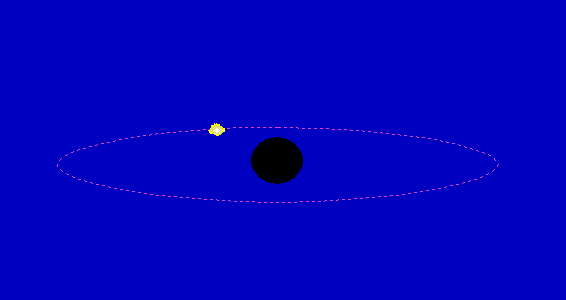This is an Astrophysical Experiment that Relativity apparently fails. Recall that Sagettarius A* has been under intense observations since 1992. Notice, to date there has been not a shred of evidence for gravitational lensing.

 Observational Evidence that Rays of Starlight Deflect only at the Plasma Limb of the Stars Finding Clearly Supported by Astrophysical Evidence Illustration: Rays of Starlight deflect at the Stellar Plasma Limb of Stars Plasma Focusing from Left to Right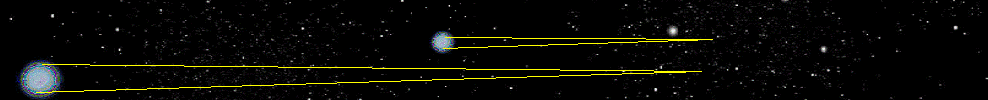Note: 1) The Plasma Focal Length = 560 AU's for Stars with Mass and Radius of Sun 2) Mean Distance between Stars in our region is ca 4 Light-Years >> 560 AU's For reasons 1) and 2) Einstein Rings in Star-filled skies are not observed
 Starlight deflected by any sun-like star with solar mass Msun and solar radius Rsun will come to a focus at 560 astronomical units (AU's). Arbitrary stars with stellar mass M and stellar radius R will have a plasma focal length of [(R/Rsun)(Msun/M)]x560 AU's. Hence, a more dens star with a solar radius Rsun will have a shorter plasma focul length < 560 AU's.
 Light From Distant Star s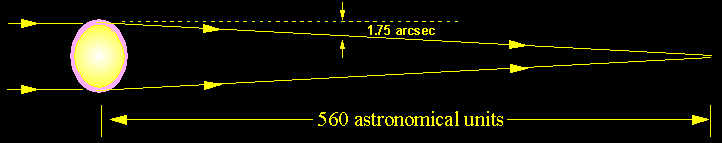ss observer seesEinstein ring  image of distant star

Solar Plasma Focal Length

 Theoretically, an observer positioned at the plasma focal length of the sun should see the image of a low impact parameter Einstein Ring of a distant star co-linearly aligned with the lensing sun and the observer.

 Plasma Focal Length as function of Star Density

Star with Twice the Density of the Sun or

 2.0 Solar Masses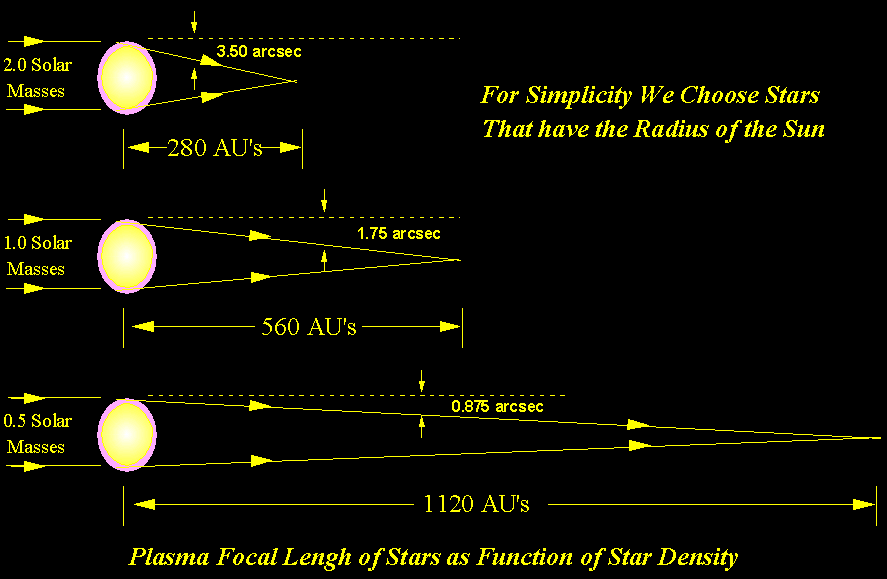Star with Density Equal to that of the Sun or

 1.0 Solar Masses

Star has Half the Density of the Sun or

 0.5 Solar Masses

Burning Question:  Where are the Einstein Rings?

Light rays from any sun-like star of solar mass Msun and solar radius Rsun will come to a focus at a distance of 560 astronomical units, assuming a gravitational deflection occurs at the plasma limb precisely at the angle of 1.752 arcsec. More Dense Stars will have Shorter Focal Lengths. Less Dense Stars will have Longer Focal Lengths.

Most importantly, ALL waves deflected by Stars could never reach the distant telescopes of an Earth-based observer as the Plasma Focal Lengths are all far less than the astronomical distances of the Earth-based Observer.

Assuming the validity of General Relativity, microwaves and starlight should be deflected at various impact parameters clearly about the plasma limb of the sun and stars. At minimum impact parameter, i.e., the distance of the nearest point on a deflected ray of microwaves within the plasma limb to center of the sun), the angle of deflection historically has been recorded to be exactly 1.752 arcsec. Since the thickness of the plasma limb is a small fraction of the solar radius, then for all practical purposes the minimum impact parameter may be considered to be Rsun.  At higher impact parameters, even in plasma-free vacuum space, General Relativity predicts reduced gravitational deflections, as illustrated in the above "alternating" animation title: The Prediction of General Relativity and the Observations Alternately.

 Prediction of General Relativity Gravitational Deflection of Microwaves vs Impact Parameter Impact Parameter Gravitational Deflection of Microwaves Rsun 1.752 arcsec Observed 2Rsun 1.752/2 = 0.876 arcsec Not Observed 3Rsun 1.752/3 = 0.584 arcsec Not Observed nRsun 1.752/n  arcsec Not Observed

The image of a low impact parameter Einstein Ring should be visible to any observer located near, but not beyond the Plasma Focal Length of a sun-like star, as illustrated above. At higher impact parameters clearly above the plasma limb of stars, it is clear from solar observations that microwave deflections do not occur in the plasma-free space clearly above the plasma limb of stars. For this very reason the gravitational lensing of a stellar plasma-limb lensing system would not be visible to observers at astronomical distances far from a stellar plasma lensing star. Plasma Limb Focusing can be observed only by placing a remote sensing system in deep space near the Plasma Focal Length of the Sun or a Star.

The gravitational deflection of light apparently does not take place anywhere in plasma-free vacuum space. An impact parameter of a deflected light ray must take place in plasma-free space as well as in the plasma limbs of the stars and the sun. As a consequence, and as supported by enormous observational evidence, the Einstein Rings can not be seen anywhere in the star-filled skies in our region of space; clearly a direct violation of the light bending rules of General Relativity.

Concepts of Mainstream, Literature and Academia

Not Observed Anywhere in Astronomy

Finding Clearly Supported by Astrophysical Evidence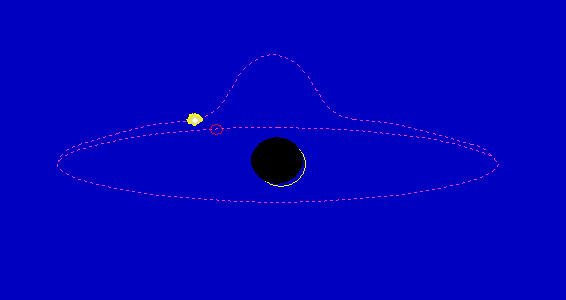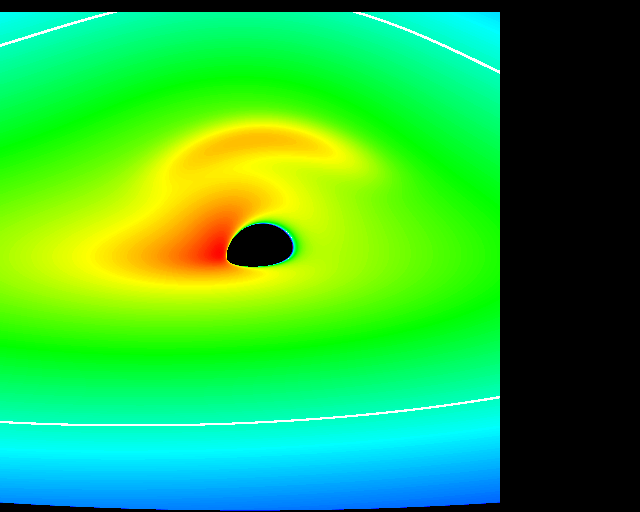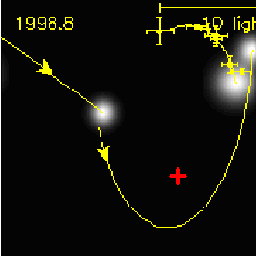SOME ANIMATED ARTIST DEPICTIONS SEEN ONLY IN THE LITERATURE

NOT SEEN ANYWHERE IN ASTRONOMY

 A CLEAR OBSERVATIONAL FACT Microwaves from extragalactic radio sources deflect only at the plasma limb of the sun and precisely at the angle of 1.752 arcsec. Deflections of these microwaves at higher impact parameters or at angles less than 1.752 arcsec have never been observed for the reasons listed above.

 II. THE FUNDAMENTALS APPLIED TO CORRECTLY DESIGNED TOOLS

We shall now correctly apply the Mathematical Physics Fundamentals to a Correctly Designed Lensing Tool. We shall now use the correctly formulated Mathematical Physics fundamentals to graphically represent the gravitational deflection of a light ray by a point-like gravitating lens according to the Gravitational light bending rule of General Relativity.

We now focus on the subject

The Critical Impact Parameter

A Mathematical Illustration

Assume we have two Observers, (a Far observer on the left and a Near observer on the right), the Lens (x) and the Source (the red * star) are co-linear and that the Lens (x) is exactly halfway between the Source * and the Far Observer.  Note that in Figure B, the axis of symmetry is exactly perpendicular to the imaginary line joining the Source * and the Far observer. Also, we may assume that all light rays emitted by the Source * belong theoretically to the same family of curves and are gravitationally bent according to the Light Bending Rule of General Relativity. We shall examine selectively 3 light rays (the rays that are labeled with color yellow). We note that only in Figures B and C the light rays (labeled yellow) will bring optical information to the telescope of the Observers, namely, the Far and the Near Observers. Figure A illustrates a selected (yellow) light ray that cannot be observed by either observer.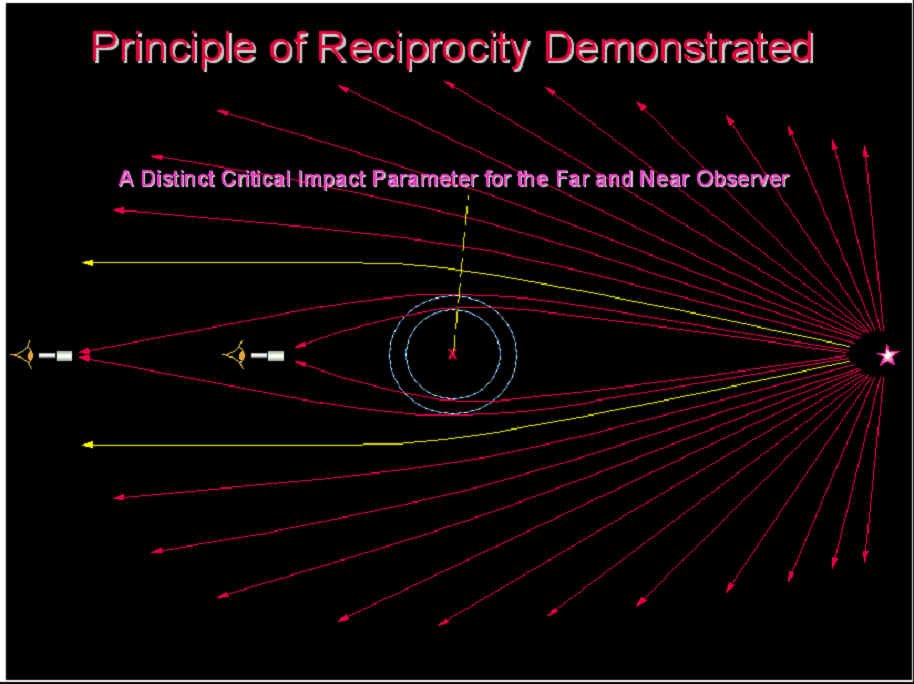Figure A  As illustrated, neither the Far (left) nor the Near (right) Observer sees the selected yellow light ray. Note the axis of symmetry leans away from both observers. Since the selected yellow light rays do not connect with an observer that is located on the imaginary line connecting the co-linearly located Source, the Lens (x) and the Observer receiving the gravitationally deflected "yellow" light, the axis of symmetry must lean away from both observers. Moreover, the gravitationally deflected rays of light that are nearly parallel with one another or found to be spreading out could never be seen by the co-linearly located observers. This is a very important fundamental of optical lensing, not found in the literature or anywhere in any text book. IMPORTANT NOTE missed by all too many researchers, professors and teachers of this subject matter. All light rays under influence of a point-like gravitational lens would theoretically have an axis of symmetry associated with that particular light ray. Let us imagine that if the axis of symmetry were to be spun around 180 degrees, where the direction of rotation were along the axis of symmetry of a gravitationally bent light ray, and assume that, for analytical purposes, the bent light ray is rigid and hypothetically attached to the spinning axis, the curved light ray would remain unchanged after having being spun around. (Hence, The curved light ray is symmetric about the axis of symmetry.)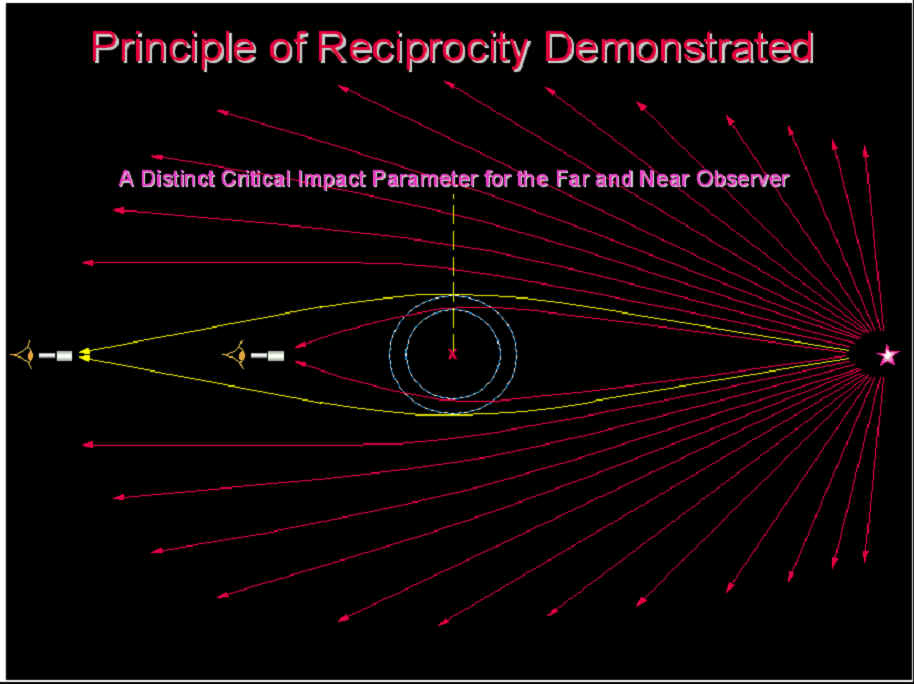Figure B  We note here the axis of symmetry for the yellow light ray that connects with the Source * and the Far Observer, which is exactly perpendicular to an imaginary line that connects the Source * and the Far Observer. The General Relativity Light Bending Rule may be considered a symmetrical principle simply because General Relativity does not distinguish between rays of light, some of which may be approaching, some of which may be receding the Lens (x). The gravitational effect acting on the light ray on approach and on receding is exactly the same. Since the Lens (x) is exactly midway between the Source on the Observer that collects the gravitationally bent light ray (yellow curve), the axis of symmetry has to be perpendicular to the imaginary line that connects the Source * and the Far Observer as illustrated above.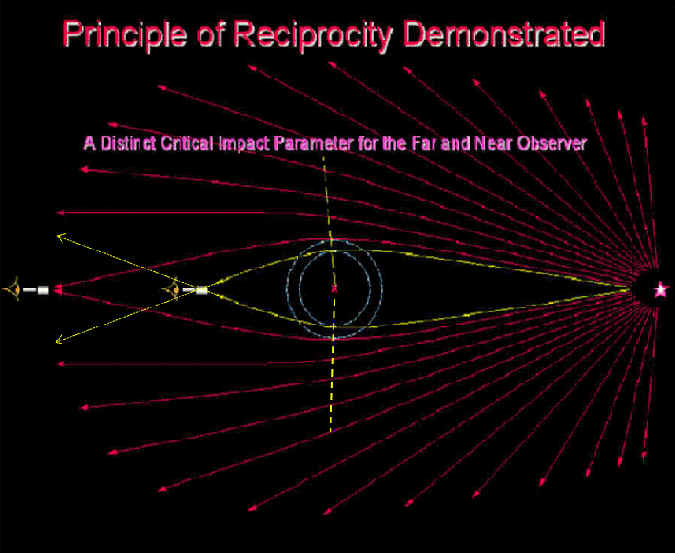Figure C  Likewise, we note here that the axis of symmetry for the yellow light ray that connects with the Source * and the Near Observer has to lean towards the Near Observer simply because the Near Observer, i.e., the observer being closest to the Lens (x), will note that the integrated effect of gravitational lensing acting on the light ray by the Lens (x) has to be greater, as this time the Lens (x) is not midway between the observer and the source. The light ray would be lensed downward below the imaginary line joining the Source * and the Near observer as illustrated in Figure C, if the light ray were to pass by the Near Observer and continue downward on a path in free space. Likewise, since this effect is a 3-Deminsional effect, which wraps around the imaginary line joining the Source and the Observers, the light ray of the bottom (yellow) curve would continue upward on a path in free space as illustrated in Figure C. This would of course assume the validity of the Light Bending Rule of General Relativity.

Mathematical Physics Fundamentals

of Gravitational Deflection Correctly Applied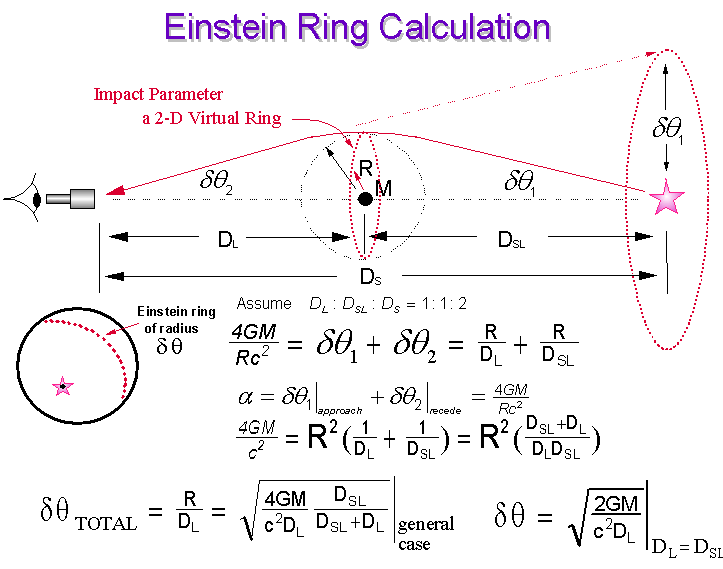Figure D  Einstein Ring Calculation; the General Case (DL ≠ DSL)

Here, the General Case (DLDSL) does not assume that the lens is midpoint between the observer and the source, as is illustrated in most textbooks.  Note that the simplified special case presented in most textbooks, assumes that  DL = DSL , where DL is the distance between the observer and the lens and DSL is the distance between the light source and the lens, placing the lens exactly halfway between the light source and the observer. This essential Mathematical Physics principle on lensing, is often totally missed by researchers attempting to deal with this topic. From symmetry requirement, the gravitational effect on a light ray upon approach to a gravitating mass positioned exactly at the midpoint of a line joining the source and the observer, must equal that of the gravitational effect on the light ray upon receding the gravitating mass, whereby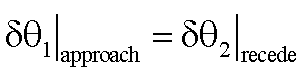and thus,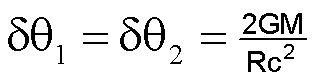and consequently the total lensing due to the total integrated effect of gravitational deflection acting on the light ray is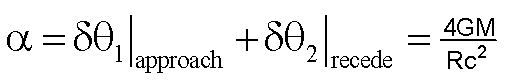For Details on this topic please click to the Significant Findings/SignificantFindings03.htm

 III. THE FUNDAMENTALS APPLIED TO A FLAWED TOOL

 A Flawed Tool due to the false teaching and/or misunderstanding of the fundamentals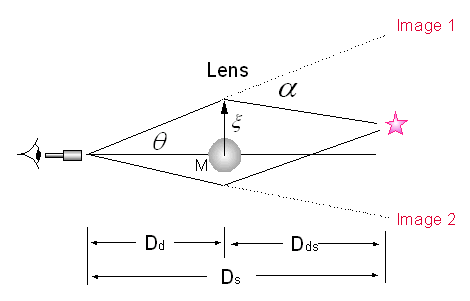Ray Geometry Technique for Gravitational Lensing Researchers utilizing this tool seek alternative simplifications for the application of the cumbersome light bending rule of General Relative and may or may not be thoroughly familiar with the basic principle of the light bending rule of General Relativity given by Equation (1),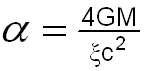(1) where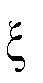is the impact parameter. In the case of microwave deflections at the solar plasma limb, this work shows that the gravitational deflections of microwaves occur only at the solar plasma limb due to minimum-energy or least-time conservations of the path of microwaves propagating in the solar plasma limb. An equating of the impact parameter and the solar radius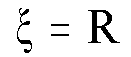(2) by the mainstream researchers dealing with this subject can only be either an innocent oversight or a deliberate deception. Equation (1) can be derived using the minimum-energy or least-time conservations of energy considerations (for details and Reference see: /details09.htm) and not simply by the use of simple, clumsy schemes of geometry such as the Ray Geometry Technique for Gravitational Lensing. The correct geometrical picture as well as the correct Mathematical Physics of the conservation of energy, the Physics of the minimum energy path or the minimum time path and the principle of reciprocity must all be considered for any correct interpretation of the observed astrophysical events dealing with gravitational lensing. From the above Figure depicting the so-called  "Ray Geometry Technique for estimating Gravitational Lensing", the derived angle of Equation (3)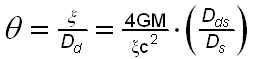(3) for estimating the gravitational deflection can not be an exact equivalence to that of General Relativity, i.e., that of Equation (1). It is readily see that, as the source approaches the vicinity of the gravitational lens, the adjustable parameter Dds , the distance between the source and the lens, gets smaller or approaches zero. Ds = 26,000 light years, where Ds is the distance between the observer and the source. Any lensing effect estimated by this geometrical "ray" scheme can only be a very poor approximation to that of General Relativity, where the derived angle given by Equation (3) will approach a very small value, i.e., zero as Dds approaches zero. This suggests incorrectly that the lensing effect would be minimized for sources located in proximity to or near to the gravitating mass or to the lens. Researchers not familiar with the Physics of gravitational lensing and the profound fundamentals thereof should not rely on such tools as the so-called "Ray Geometry Technique" used for the purpose of estimating Gravitational Lensing without taking the painstaking effort to apply the Profound Fundamentals of the phenomenon under observation.

 False Alarms due to Misinterpretation of the Observations Use of the Flawed Designed Tools Incorrect Application of the Fundamentals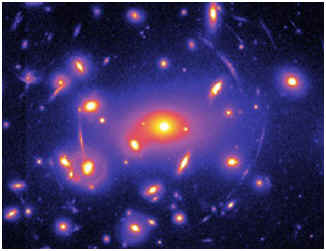DETAILS: Click on the Link:  /SignificantFindings08_B.htm or Simply Click Above on the Image of the False Lensing Galaxy

A SUBJECT MAINSTREAM PHYSICS FAILS TO TEACH

AND IS EMBARRASSED TO TALK ABOUT

 FUNDAMENTALS and FACTS the Academia, the Literature and the Referees are getting completely WRONGDr. Albert Einstein's own words:  "Wenn die Lichtgeschwindigkeit auch nur ein bißchen von der Geschwindigkeit der Lichtquelle abhängig ist, dann ist meine ganze Relativitätstheorie und Gravitationstheorie falsch." {Zitat A. Einsteins von einem Brief an Erwin Finley-Freundlich: August 1913} "If the velocity of light is only a tiny bit dependent on the velocity of the light source, then my whole theory of Relativity and Gravitation is false." {Quotation of A. Einstein from a letter to Erwin Finley-Freundlich: August 1913}.

The Challenge